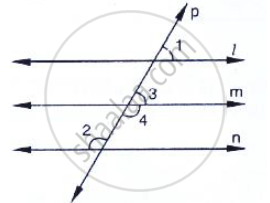# In the Below Fig, If L || M || N and ∠1 = 60°, Find ∠2. - Mathematics

In the below fig, if l || m || n and ∠1 = 60°, find ∠2.#### Solution

Since l parallel to m and 𝑝 is the transversal

∴Given: l || m || n, ∠1 = 60°

To find ∠2

∠1 = ∠3 = 60°                 [Corresponding angles]

Now,

∠3 and ∠4 are linear pair of angles

∠3 + ∠4 = 180°

60° + ∠4 = 180°

∠4 = 180° - 60°

∠4 = 120°

Also, m || n and P is the transversal

∴∠4 = Ð2 =120°                        [Alternative interior angle]

Hence ∠2 =120°

Concept: Parallel Lines and a Transversal
Is there an error in this question or solution?

#### APPEARS IN

RD Sharma Mathematics for Class 9
Chapter 10 Lines and Angles
Exercise 10.4 | Q 10 | Page 47